In this article, we are going to make a Sun Tracking Solar Panel using Arduino, in which we will use two LDRs (Light-dependent resistor) to sense the light and a servo motor to automatically rotate the solar panel in the direction of the sunlight. The advantage of this project is that the Solar panels will always follow the sunlight will always face the sun to get charge all the time and can provide the supply the maximum power. The prototype is very easy to build. Below you will find the complete description of how it works and how the prototype is made.

### Required Components for Arduino Solar Tracker:

The following are the component requires to build a solar tracking system using Arduino, most of the components should be available in your local shop.

• Servo Motor (sg90)
• Solar panel
• Arduino Uno
• LDR’s X 2 (Light Dependent Resistor)
• 10K resistors X 2
• Battery (6 to 12V)

### How does a single axis solar tracker work?

In this project, LDR’s are working as light detectors. Before we go into detail, we will have to understand how the LDR’s work. LDR (Light Dependent Resistor) also known as photo resistor is the light sensitive device. Its resistance decrease when the light falls on it and that’s why it is frequently used in Dark or Light Detector Circuit. Check the various circuits based on LDR here.

The two LDR’s are placed at the two sides of the solar panel and the Servo Motor is used to rotate the solar panel. The servo will move the solar panel towards the LDR whose resistance will be low, mean towards the LDR on which light is falling, that way it will keep following the light. And if there is some amount of light falling on both the LDR, then the servo will not rotate. The servo will try to move the solar panel in the position where both LDR’s will have the same resistance means where the same amount of light will fall on both the resistors and if the resistance of one of the LDR will change then it rotates towards lower resistance LDR. Check the Demonstration Video at the end of this Article.

### How to build a rotating solar panel using Arduino:

To make the prototype, you will have to follow the below steps:

Step 1:

First of all, take a small piece of cardboard and make a hole at one end. We will insert the screw in it to fix it with the servo later on.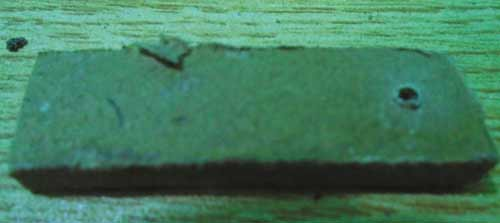Step 2:

Now fix two small pieces of cardboard with each other in a V shape with help of glue or hot gun and place solar panel on it.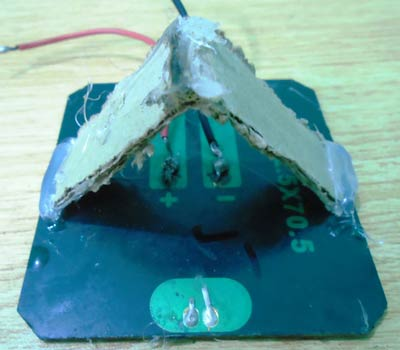Step 3:

Then attach the bottom side of the V shape to the other end of small piece of cardboard in which you made a hole in first step.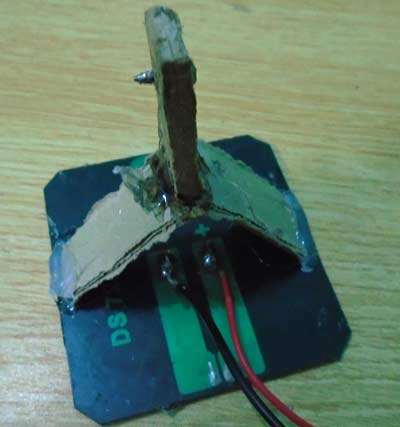Step 4:

Now insert the screw in the hole you made on card board and insert it through the hole into the servo. The screw comes with the servo motor when you buy it.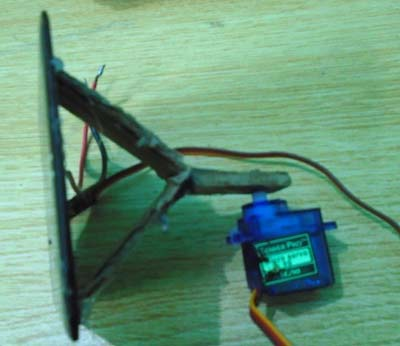Step 5:

Now place the servo on another piece of cardboard. The size of the cardboard should be larger enough so that you can place a Arduino Uno, a breadboard and a battery on it.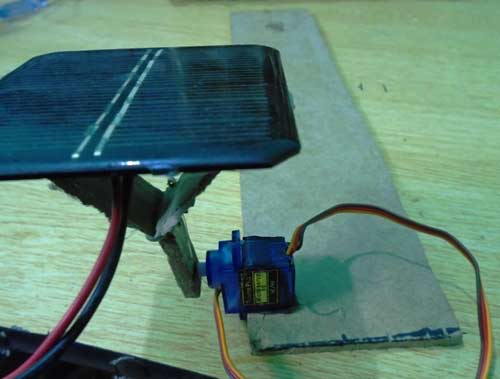Step 6:

Attach the LDRs on the two sides of the solar panel with the help of glue. Make sure you have soldered the wires with the legs of the LDR’s. You will have to connect these with the resistors later on.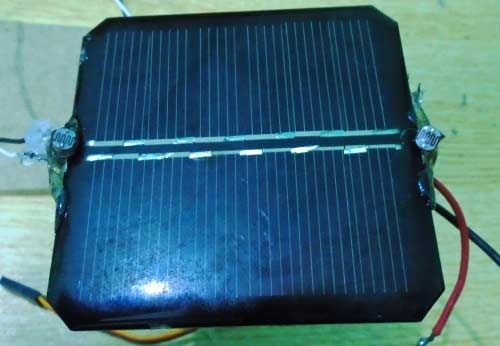Step 7:

Now place the Arduino, battery and the breadboard on the cardboard and make the connection as described in the Circuit diagram and Explanation section below.  The final prototype is shown below.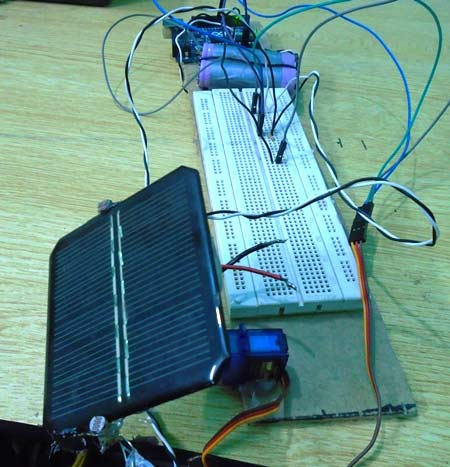### Circuit Diagram and Explanation:

The complete circuit diagram for the solar tracking arduino project is shown below. As you can see the circuit is very simple and can easily be built with help of a small breadboard.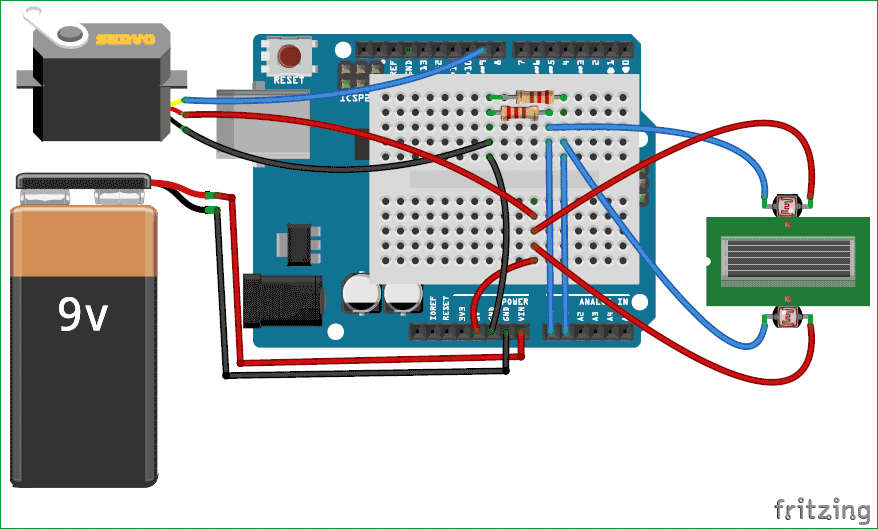In this Arduino Solar Panel Tracker, Arduino is powered by the 9V battery and all the other parts are powered by the Arduino. Arduino recommended input voltage is from 7 to 12 volts but you can power it within the range of 6 to 20 volts which is the limit. Try to power it within the recommended input voltage. So connect the positive wire of the battery to the Vin of the Arduino and the negative wire of the battery to the ground of the Arduino.

Next, connect the servo to the Arduino. Connect the positive wire of the servo to the 5V of Arduino and ground wire to the ground of the Arduino and then connect the signal wire of Servo to the digital pin 9 of Arduino. The servo will help in moving the solar panel.

Now connect the LDRs to the Arduino. Connect one end of the LDR to the one end of the 10k resistor and also connect this end to the A0 of the Arduino and connect the other end of that resistor to the ground and connect the other end of LDR to the 5V. Similarly, connect the one end of the second LDR to the one end of the other 10k resistor and also connect that end to the A1 of Arduino and connect the other end of that resistor to the ground and connect the other end of LDR to 5V of Arduino.

### Single-axis solar tracker using Arduino code:

Code for this Arduino based Solar Panel Tracker is easy and well explained by comments. First of all, we will include the library for servo motor. Then we will initialize the variable for the initial position of the servo motor. After that, we will initialize the variables to read from the LDR sensors and Servo.

```#include <Servo.h>      //including the library of servo motor
Servo sg90;                   //initializing a variable for servo named sg90
int initial_position = 90;    //Declaring the initial position at 90
int LDR1 = A0;                //Pin at which LDR is connected
int LDR2 = A1;                //Pin at which LDR is connected
int error = 5;                //initializing variable for error
int servopin=9;```

sg90.atach(servopin) command will read Servo from the pin 9 of Arduino. Next, we set the LDR pins as input pins so that we can read the values from the sensors and move the solar panel according to that. Then we set the servo motor at 90 degree which is the initial position for the servo.

```void setup()
{
sg90.attach(servopin);  // attaches the servo on pin 9
pinMode(LDR1, INPUT);   //Making the LDR pin as input
pinMode(LDR2, INPUT);
sg90.write(initial_position);   //Move servo at 90 degree
delay(2000);                   // giving a delay of 2 seconds
}```

Then we will read the values from the LDRs and will save in R1 and R2. Then we will make the difference between the two LDRs to move the servo accordingly. If the difference between them will be zero that it means that the same amount of light is falling on both the LDR’s so the solar panel will not move. We have used a variable named error and its value is 5, the use of this variable is that if the difference between the two LDRs will be under 5 then the servo will not move. If we will not do this then the servo will keep on rotating. And if the difference is greater than error value (5) then the servo will move the solar panel in the direction of the LDR, on which light is falling. Check the Full Code and demo Video below.

```  int R1 = analogRead(LDR1); // reading value from LDR 1
int diff1= abs(R1 - R2);   // Calculating the difference between the LDR's
int diff2= abs(R2 - R1);

if((diff1 <= error) || (diff2 <= error)) {
//if the difference is under the error then do nothing
} else {
if(R1 > R2)
{
initial_position = --initial_position;  //Move the servo towards 0 degree
}
if(R1 < R2)
{
initial_position = ++initial_position; //Move the servo towards 180 degree
}
}

```

So that is how you can build a simple Solar Panel Tracker, which will automatically move towards the light like a sunflower. Here we have used the low power solar panel to reduce the weight, if you are planning to use high power or heavy solar panel then you need to choose the Servo motor accordingly.

Code

/*

Arduino solar tracker code

www.circuitdigest.com

*/

#include <Servo.h>      //including the library of servo motor
Servo sg90;             //initializing a variable for servo named sg90
int initial_position = 90;   //Declaring the initial position at 90
int LDR1 = A0;          //Pin at which LDR is connected
int LDR2 = A1;          //Pin at which LDR is connected
int error = 5;          //initializing variable for error
int servopin=9;
void setup()

sg90.attach(servopin);  // attaches the servo on pin 9
pinMode(LDR1, INPUT);   //Making the LDR pin as input
pinMode(LDR2, INPUT);
sg90.write(initial_position);   //Move servo at 90 degree
delay(2000);            // giving a delay of 2 seconds
}

void loop()

int diff1= abs(R1 - R2);   // Calculating the difference between the LDR's
int diff2= abs(R2 - R1);

if((diff1 <= error) || (diff2 <= error)) {
//if the difference is under the error then do nothing
} else {
if(R1 > R2)
{
initial_position = --initial_position;  //Move the servo towards 0 degree
}
if(R1 < R2)
{
initial_position = ++initial_position; //Move the servo towards 180 degree
}
}
sg90.write(initial_position); // write the position to servo
delay(100);
}

Video

Subscribe below to receive most popular news, articles and DIY projects from Circuit Digest

### Related Content

•decidechari
Mar 02, 2017

i m looking for wireless power transfer adurino based projects code and prototype

•vikas bhandi
Mar 18, 2017

i have done all the connection as shown in circuit diagram but still servo motor is running too slow

•hanzla
May 30, 2017

#include <Servo.h>
Servo myservo; // create servo object to control a servo
int sensorOne=2; //digital pin 2 has connected to the resistor of first sensor
int sensorTwo=3; //digital pin 3 has connected to the resistor of second sensor
int inputOne=0; //variable to store the values from the analog pin 0
int inputTwo=1; //variable to store the values form the analog pin1
int currPos=0; // variable to store the servo position
void setup()
{
pinMode(sensorOne,OUTPUT); //initialize the digital pin as output
pinMode(sensorTwo,OUTPUT); //initialize the digital pin as output
digitalWrite(sensorOne,HIGH);
digitalWrite(sensorTwo,HIGH);
myservo.attach(9); // attaches the servo on pin 9 to the servo object
myservo.write(currPos); // tell servo to go to position in variable 'pos'
}
void loop()
{
int diff=valueOne-valueTwo; //difference of two values from the sensors
if(valueOne<950 || valueTwo<950) //if light is present
{
if(diff>=100) //if light source is towards right
{
currPos=currPos+2;
myservo.write(currPos); // tell servo to go to position in variable 'pos'
delay(30); // waits 30ms for the servo to reach the position
}
else if(diff<=-100) //if light source is towards left
{
currPos=currPos-2;
myservo.write(currPos); // tell servo to go to position in variable 'pos'
delay(30); // waits 30ms for the servo to reach the position
} //end of inner if block
} //end of main if block
} //end of void loop() method

•vignesh
Oct 22, 2017

this code is correct

•rashid
Feb 15, 2018

this code for what use

•uhorchuk
Mar 20, 2017

What code is for 4 actuators (optional rotating vertical)?

•Imran
Sep 12, 2017

Sir i want ardino program can u please mail to me

•sumanth
Sep 19, 2017

i have done all the connections but the servo is not moving according to the ldr inputs .please help me

•yuvraj
Oct 22, 2017

sir how can I write initial position at 90 degree please write the last code for initial position

•Md.Kamrojjaman
Nov 30, 2017

Please anybody send me this circuit diagram

•Akash
Dec 12, 2017

I have a solar panel of 100watts,also it is 5kg in weight so can you just help me with choosing the rating of servo motor so that it can rotate the panel.

•Prathima Ayinala
Jan 03, 2018

pleasse any body send me circuit diagram for this
it's urgent

•soniya
Feb 06, 2018

will this project complete with in a month
send the requirements
code
circuit diagram

• AISHA
Feb 07, 2018

Everything that you asked is given above. Can it be completed? it depends on you

•Sridhara Vignesh
Feb 16, 2018

hy I'm a college student 1st year ,can u give me a clear picture of how to solder the ldrs to the solar panel?

•Jitesh
Mar 16, 2018

I want to make that with dc motor then what is the code with dc motor.

•Saurav
Apr 26, 2018

Please mail me the circuit diagram

•Tejassvi Chopraa
May 25, 2018

10K Ohm resistor but how many watt?

•mansoor shah
Jul 20, 2018

hi

Dear sir i use 12 volt recycle car wiper motor for solar stand moving they have 2 wires and yours circuits have a servo motor which have 3 wires what can i do to installed my motor

• Aswinth Raj
Jul 23, 2018

Using a Wiper motor to control the solar pannel is a bad idea. Because wiper motor does not have position control? Why did you not use a servo? Is you panel very big for a servo?

• Karan Suraiya
Oct 03, 2019

For me the code is working but when the ldr senses light it keeps rotating the servo continuosly and doesnt stop

• taran
Oct 03, 2019

could you make this using a stepper motor 28BYJ-48 (used to turn the whole thing) with a solar panel, photo-resistors on a piece of cardboard on a pole-like thing??

• Jes
Oct 03, 2020

can someone help me here?